# Doppler Effect

Doppler effect is a common phenomenon that we observe in our everyday lives. For instance, if you are standing on the footpath, you hear a police vehicle approaching in the distance. First, the sound of the siren is faint and it gets louder as the vehicle reaches closer to you. Once the police car crosses you and goes ahead the sound gradually decreases again. This effect is basically known as the Doppler effect.

Generally, the doppler effect can be defined as the change in wave frequency (whether it is light or sound) during relative motion between the source of the wave and the observer. This effect can be observed every time a source wave is moving in relation to the observer. The Doppler effect was discovered in the year 1842 by an Austrian mathematician and physicist named Christian Johann Doppler. The effect was named after him.

## What is Doppler Effect?

When the source and observer are moving relative to each other, the frequency observed by the observer (fa) is different from the actual frequency produced by the source (f0). This is basically the definition of the Doppler effect in Physics. Here, when the source of waves is moving towards the observer they will have an upward shift in frequency. As for the observers from whom the source is receding, there will be a downward shift in frequency.

However, it should be noted that the effect does not occur as a result of the actual change in the frequency of the wave source. The Doppler effect can be observed in both sound waves and light waves. While we have already described the effect in sound waves, the following is an example of the Doppler effect for light waves.

If we observe the light of a star from earth it usually shifts toward the red end of the spectrum (lower frequency or longer wavelength) when the earth and star are moving away from each other. This is also known as red-shift. On the other hand, it tends to move toward the violet side (higher frequency or shorter wavelength) if both earth and the star are coming close to each other. This is also called the blue shift. As a result of this phenomenon, the doppler effect is used in studying the motion of stars. It is also an integral part of modern theories of the universe.

#### Doppler Effect Video Lesson### Why Do We Experience Doppler Effect?

The main reason that we experience the Doppler effect is that as the wave source moves toward the observer, each new wave crest that is formed from the source is emitted from a location that is closer to the observer. Therefore, as the source moves closer and closer the waves will now take less time to reach the observer or the time between the arrivals of new wave crests is reduced. This further causes an increase in frequency. Similarly, when the source of waves is going away, the waves are emitted from a farther location thus increasing the arrival time between each new wave. This leads to a reduction in frequency.

Nonetheless, from what we have learnt above, we can summarize that the Doppler effect could result from several factors such as;

• the motion of the observer
• the motion of the source
• the motion of the medium.

This is mainly true for sound waves. Whereas, for waves that can travel in any medium, such as light, we need to consider only the relative difference in velocity between the source and the observer.

## Doppler Effect Formula / Equation

The general form of the Doppler Effect formula is expressed as:

$$\begin{array}{l}f = \left ( \frac{c\pm v_{r}}{c\pm v_{s}} \right ) f_{o}\end{array}$$

C = propagation speed of waves in the medium;

Vr = speed of the receiver relative to the medium, +c if the receiver is moving towards the source, -c if the receiver is moving away.

Vc = speed of the source relative to the medium, +c if the source is moving away -c if the source is moving towards the receiver.

There is the main Doppler effect equation. However, this equation can change in different situations. It is adjusted or modified depending on the velocities of the observer or the source of the sound. We will see the different Doppler effect formulas in several cases or situations.

Also Read: Wave Motion

## Different Cases of Doppler Effect

When we say source and observer are moving relative to each other, we get different cases as follows.

Case I: An observer moving away from the stationary source.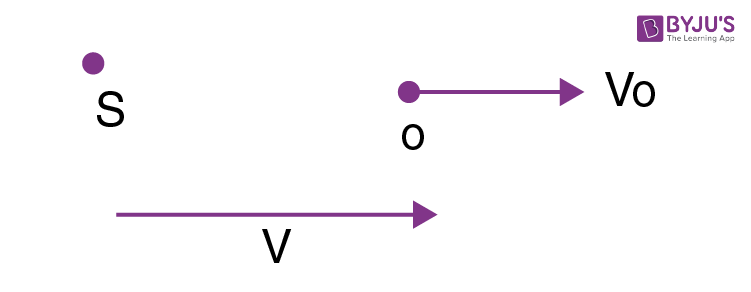Case II: An observer moving towards the stationary source.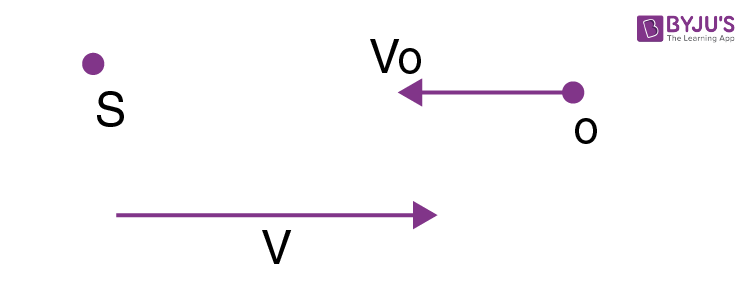Case III: A source moving away from the stationary observer.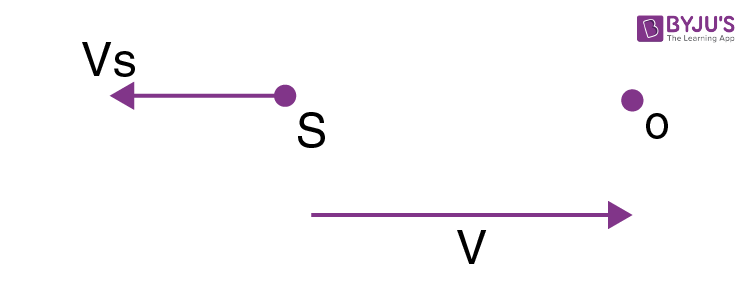Case IV: A source moving towards the stationary observer.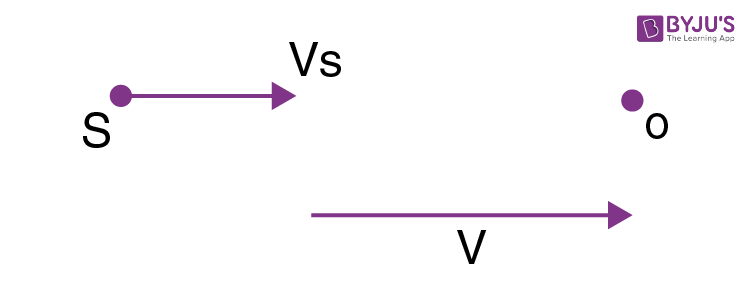Case V: A source and an observer moving towards each other.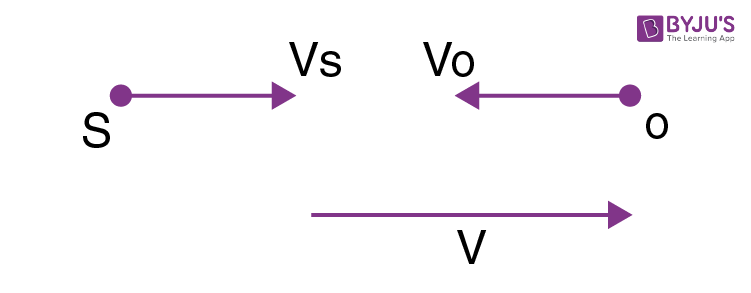Case VI: A source and an observer moving away from each other.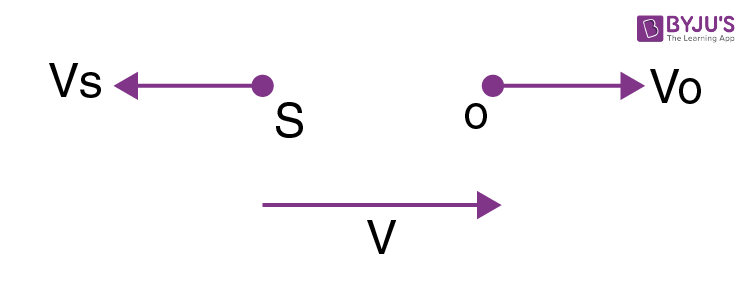Case VII: A source and an observer moving in the same direction along the direction of the velocity of sound (v).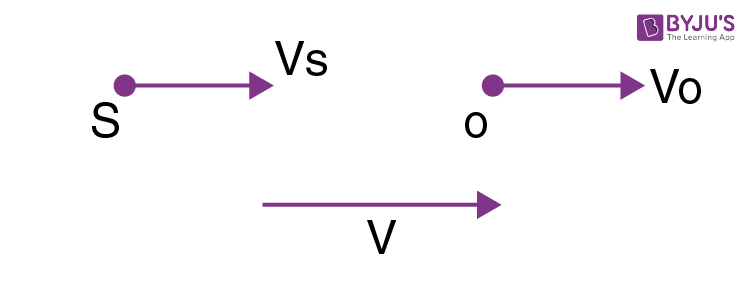Case VIII: A source and an observer moving in the same direction opposite to the direction of the velocity of sound (v)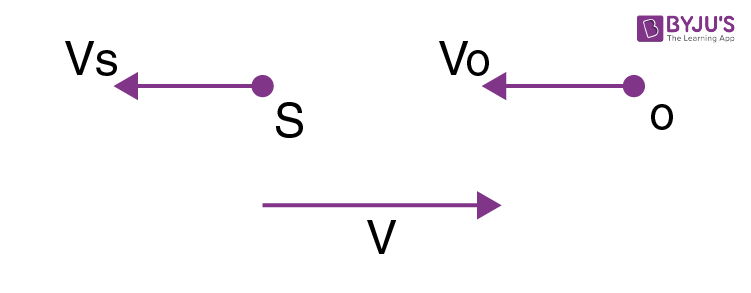When the source and observer are relatively at rest with respect to each other, then the frequency heard by the observer is equal to the actual frequency produced by the source.

fa = fo

Also Read: Superposition of Waves

In this situation, the number of cycles passing through the point of observer remains constant with time. As the observer moves towards the source (or) source moves towards the observer, the observer experiences sound waves in a relatively lesser time as the relative separation decreases. So from the formula

$$\begin{array}{l}F=\frac{1}{T}\end{array}$$
, the observer experiences a frequency more than the actual frequency produced by the source

$$\begin{array}{l}\Rightarrow \,{{f}_{a}}>{{f}_{o}}\end{array}$$

As the observer moves away from the source (or) source moving away from the observer, the observer hears sound waves after a relatively long time as the relative separation increases, so from the formula

$$\begin{array}{l}F=\frac{1}{T}\end{array}$$
the observer experiences a frequency less than the actual frequency produced by the source.

$$\begin{array}{l}\Rightarrow \,{{f}_{a}}<{{f}_{o}}\end{array}$$

Considering,

Case I: In this case, the observer is moving away from a stationary source. It implies that

$$\begin{array}{l}{{f}_{a}}<{{f}_{o}}\end{array}$$
$$\begin{array}{l}\Rightarrow \,{{f}_{a}}=k\,{{f}_{o}}\,\,\,\,\,\,\,\,\,\,where\,(k<1)\end{array}$$

Let k be a constant which is less than 1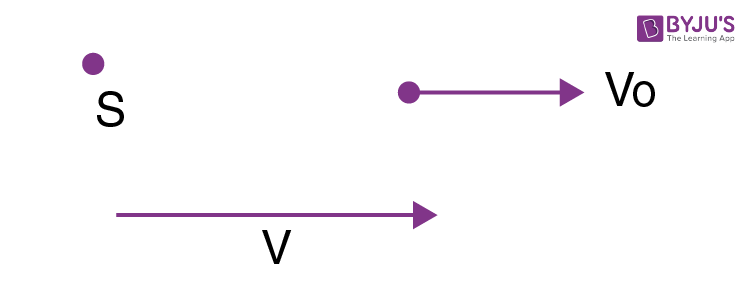The relative velocity of sound with respect to moving observer is v – vo.

$$\begin{array}{l}\Rightarrow \,\,\,\,V-{{V}_{o}}\,<\,v\end{array}$$

Therefore,

$$\begin{array}{l}\,\,\,\,\,\,\,\,\,\frac{v-{{v}_{o}}}{v}<1\end{array}$$

Comparing this with k < 1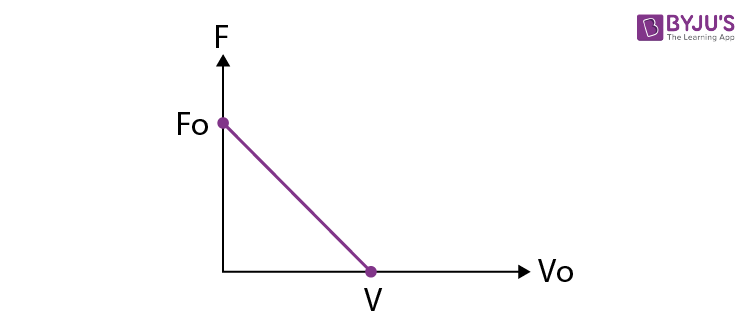We get,

$$\begin{array}{l}{{f}_{a}}=\left( \frac{V-{{V}_{O}}}{V} \right){{f}_{o}}\end{array}$$

Case II: In this case, the observer is moving towards the stationary source, it implies that

Fa > Fo

$$\begin{array}{l}\Rightarrow \,\,\,{{F}_{a}}=k{{F}_{o}}\,\,where\,\,(k>1)\end{array}$$
.

Let k is a constant which is greater than 1.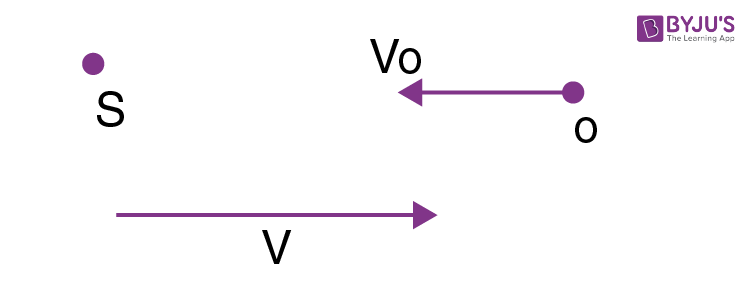The relative velocity of sound with respect to a moving observer is V + Vo.

$$\begin{array}{l}\Rightarrow \,v+{{v}_{o}}>v\end{array}$$

Therefore,

$$\begin{array}{l}\,\,\,\,\frac{V+Vo}{V}>1\end{array}$$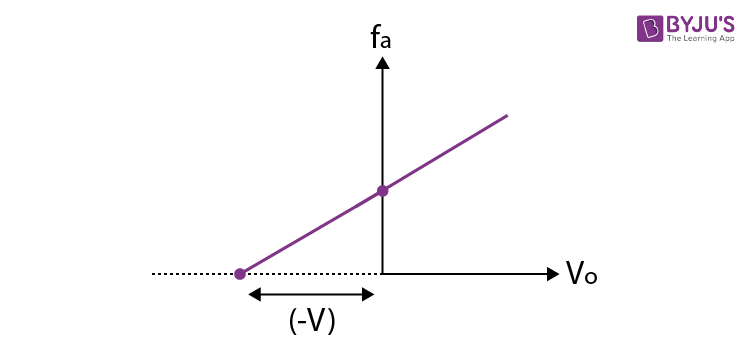Comparing this with (k > 1)

We get,

$$\begin{array}{l}{{f}_{a}}=\left( \frac{V+{{V}_{O}}}{V} \right){{f}_{o}}\end{array}$$

Case III: In this case, source moving away from the stationary observer. It implies that,

Fa < fo

$$\begin{array}{l}\Rightarrow \,\,fa=k\,fo\,\,\,\,\,\,\,\,\,\,where\,(k<1)\end{array}$$

Let k be a constant which is less than 1.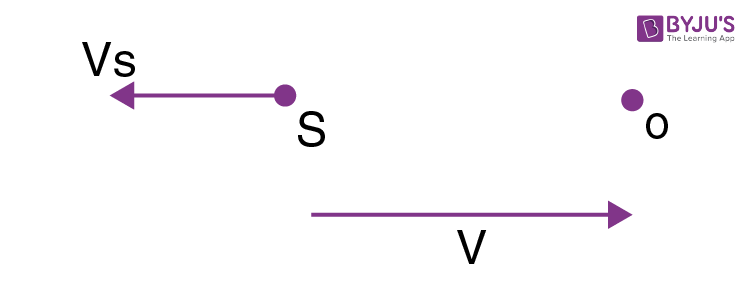The relative velocity of sound with respect to moving source is V + Vs

$$\begin{array}{l}\Rightarrow \,\,V+Vs>V\end{array}$$

Therefore,

$$\begin{array}{l}\,\,\,\,\frac{V}{V+Vs}<1\end{array}$$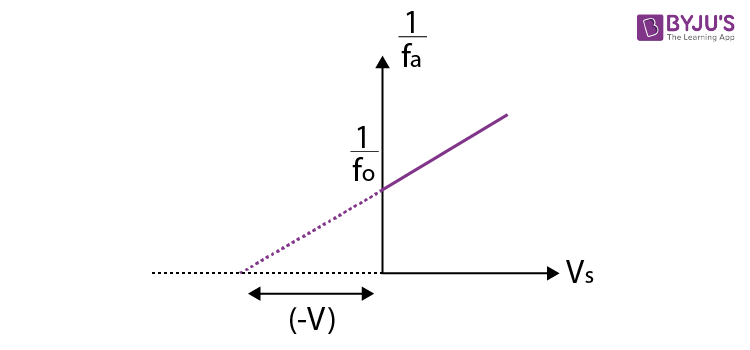Comparing this with k < 1

We get,

$$\begin{array}{l}{{f}_{a}}=\left( \frac{V}{V+Vs} \right){{f}_{o}}\end{array}$$

Case IV: In this case, the source is moving towards the stationary observer. It implies that

Fa > f

$$\begin{array}{l}\Rightarrow \,\,fa=k\,fo\,\,\,\,\,\,\,\,\,\,where\,(k>1)\end{array}$$

Let k be a constant which is greater than 1.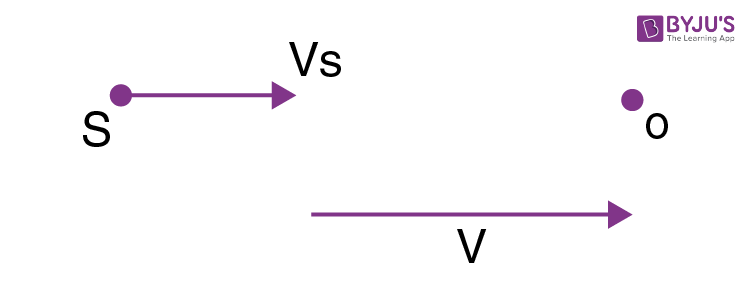The relative velocity of sound with respect to moving source is V – Vs

$$\begin{array}{l}\Rightarrow \,\,\,V-Vs<V\end{array}$$

Therefore,

$$\begin{array}{l}\,\,\,\,\,\frac{V}{V-Vs}>1\end{array}$$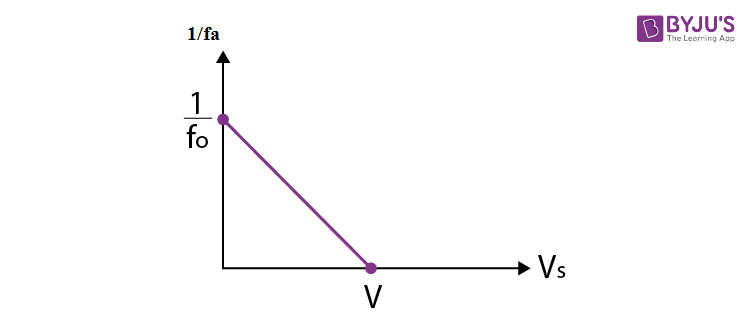Comparing this with (k > 1)

We get,

$$\begin{array}{l}{{f}_{a}}=\left( \frac{V}{V-Vs} \right)fo\end{array}$$

Case V: In this case, source and observer are moving towards each other that implies Fa > fo

(or) fa = kfo where (k > 1)

Let k be a constant which is greater than 1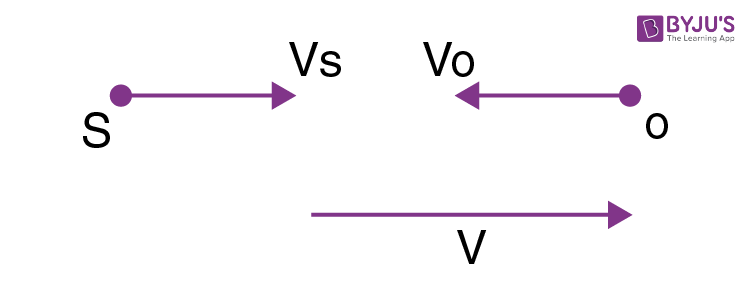The relative velocity of sound with respect to the moving source is V – Vs.

The relative velocity of sound with respect to the moving observer is V + Vo.

$$\begin{array}{l}\Rightarrow \,\,\,V-Vs<V\,\,and\,\,V+Vo>V\end{array}$$
$$\begin{array}{l}\,\,\,\frac{V}{V-Vs}>1\,\,\,\,\,\,\,\,and\,\,\frac{V+Vo}{V}>1\end{array}$$

From above we can say that

$$\begin{array}{l}\frac{V}{V-Vs}\times \frac{V+Vo}{V}>1\end{array}$$
$$\begin{array}{l}\Rightarrow \,\,\frac{V+Vo}{V-Vs}>1\end{array}$$

Comparing this with k > 1

We get,

$$\begin{array}{l}{{f}_{a}}=\left( \frac{V+Vo}{V-Vs} \right)fo\end{array}$$

Case VI: In this case, source and observer are moving away from each other which implies that, Fa < Fo

(or) Fa = KFo where (K < 1)

Let k be a constant which is less than 1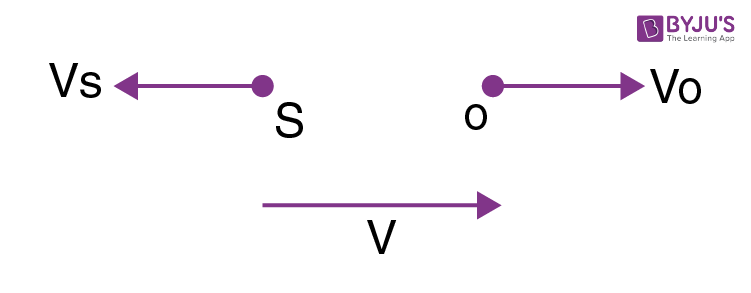The relative velocity of sound with respect to the moving source is V + Vs.

The relative velocity of sound with respect to moving observer is V – Vo.

$$\begin{array}{l}\Rightarrow \,\,\,\,V+Vs>V\,\,\,\,\,\,\,and\,\,\,\,V-Vo<V\end{array}$$
$$\begin{array}{l}\,\,\,\,\,\frac{V+Vs}{V}>1\,\,\,\,\,\,\,\,\,and\,\,\,\,\frac{V-Vo}{V}<1\end{array}$$

From above we can say that,

$$\begin{array}{l}{}^{\frac{V-Vo}{{V}}}/{}_{\frac{V+Vs}{{V}}}\,\,\,<1\end{array}$$
$$\begin{array}{l}\frac{V-Vo}{V+Vs}<1\end{array}$$

Comparing this with k < 1

We get,

$$\begin{array}{l}Fa=\left( \frac{V-Vo}{V+Vs} \right)Fo\end{array}$$

Case VII: in this case, both source and observer are moving in the same direction along the direction of the velocity of sound (v).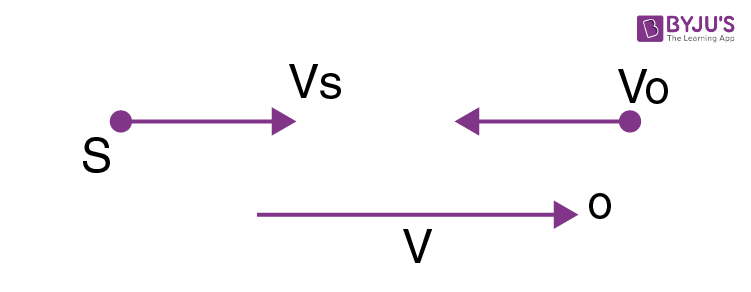When both are moving in the same direction, if suddenly the observer comes to rest then the source will be moving towards the observer.

$$\begin{array}{l}\Rightarrow Fa=\left( \frac{V}{V-Vs} \right)Fo\,\,\,\,\,\to \,\,(a)\end{array}$$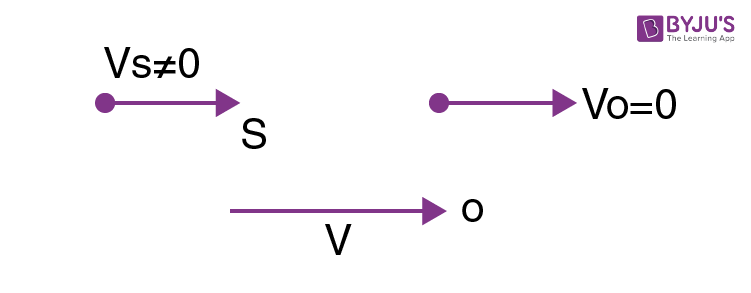When both are moving in the same direction, if suddenly the source comes to rest, then the observer will be moving away from the source.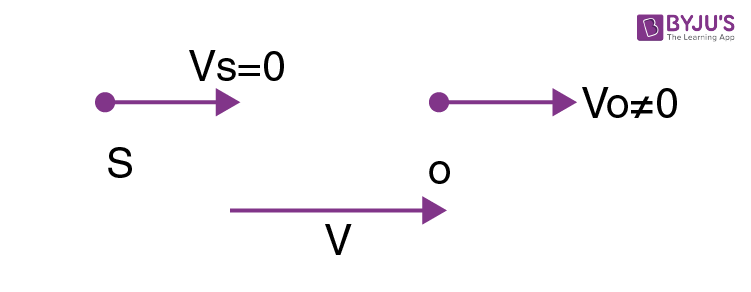$$\begin{array}{l}\Rightarrow \,\,Fa=\left( \frac{V-Vo}{V} \right)Fo\to (b)\end{array}$$

Combining (a) and (b) we get

$$\begin{array}{l}{{F}_{a}}=\left( \frac{V-Vo}{V=Vs} \right)Fo\end{array}$$

Case VIII: in this case, both source and observer are moving in the same direction but opposite to the direction of the velocity of sound (v).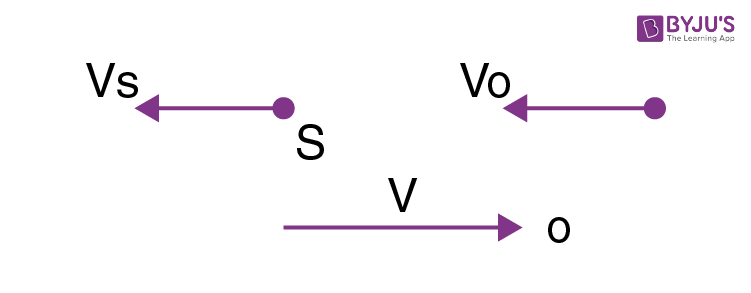When both are moving in the same direction, if suddenly observer comes to rest, then the source will be moving away from the observer.$$\begin{array}{l}\Rightarrow Fa=\left( \frac{V}{V+Vs} \right)Fo\,\,\,\,\,\to \,\,(a)\end{array}$$

When both are moving in the same direction, if suddenly the source comes to rest, then the observer will be moving towards the source.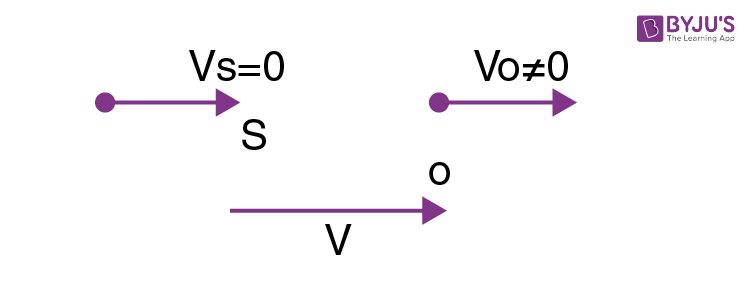$$\begin{array}{l}\Rightarrow \,\,Fa=\left( \frac{V+Vo}{V} \right)Fo\to (b)\end{array}$$

Also Read: Longitudinal and Transverse Waves

## Effect Of Motion Of Medium On Apparent Frequency

Till now we assumed medium at rest, if the medium is in motion, then two cases arise

(i) When medium moves from source to observer:

If the wind is blowing with speed Vw from the source to the observer that is in the direction of the velocity of sound, then the velocity of sound v is taken as V + Vw in all the above formulae which are derived taking medium at rest.

(ii) When medium moves from observer to source:

If the wind blows with a velocity V – Vw in all the above formulae derived taking medium at rest.

## Special Cases

Case I: Doppler’s effect in reflected sound

Consider a source of sound moving towards a stationary wall with a speed Vs emitting a sound of frequency fo. To a stationary observer o, the apparent frequency fa of sound heard by the observer directly from the source is

$$\begin{array}{l}Fa=\left( \frac{V}{V+Vs} \right)Fo\end{array}$$

To find the frequency of the reflected sound heard by the observer, assuming the image of the source s1 is the wall, then the apparent frequency is,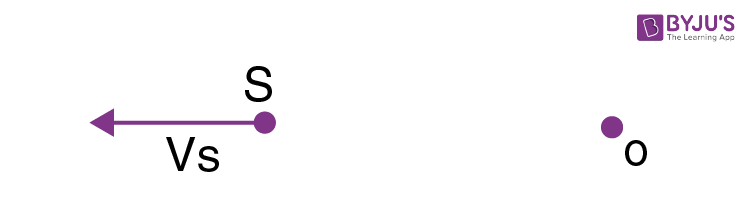$$\begin{array}{l}F{{a}^{1}}=\left( \frac{V}{V-Vs} \right)Fo\end{array}$$

Beat frequency heard by the observer is,

$$\begin{array}{l}F=F{{a}^{1}}-Fa=\left( \frac{V}{V-Vs}-\frac{V}{V+Vs} \right)Fo\end{array}$$
$$\begin{array}{l}F=\left( \frac{2VVs}{{{V}^{2}}-V_{s}^{2}} \right)Fo\end{array}$$

Case II: Sound source and observer are moving in a direction making an angle with the line joining them.

Till now we discussed only the cases in which source and observer are along the same line and joining them. If the motion is along some other direction, join source and observer by a straight line then the components of velocities along the line joining them must be considered.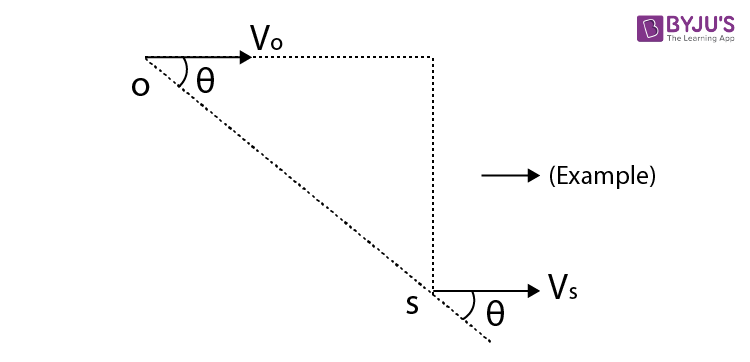For example, from the figure,

$$\begin{array}{l}Fa=\left( \frac{V+Vo\cos \theta }{V+Vo\sin \theta } \right)Fo\end{array}$$

Case III: Source (or) observer rotating:

1. When the source is rotating:

When the source moves towards the observer, then the frequency heard is maximum

$$\begin{array}{l}{{F}_{\max }}=\left( \frac{V}{V-Vs} \right)Fo\end{array}$$

When the source is moving away from the observer, the frequency heard is minimum.

$$\begin{array}{l}{{F}_{\min }}=\left( \frac{V}{V+Vs} \right)Fo\end{array}$$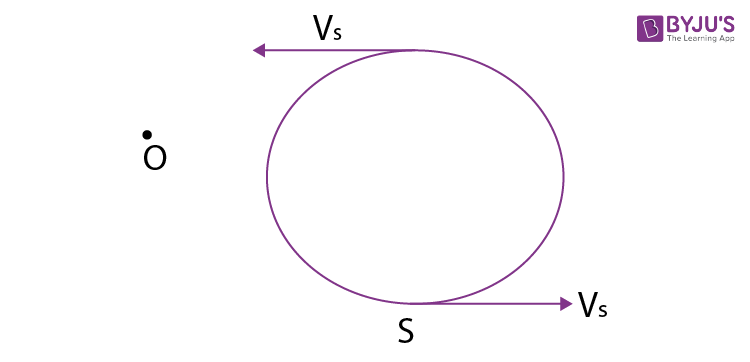If the observer is situated at the centre of rotation then there will be no change in the frequency of the sound heard.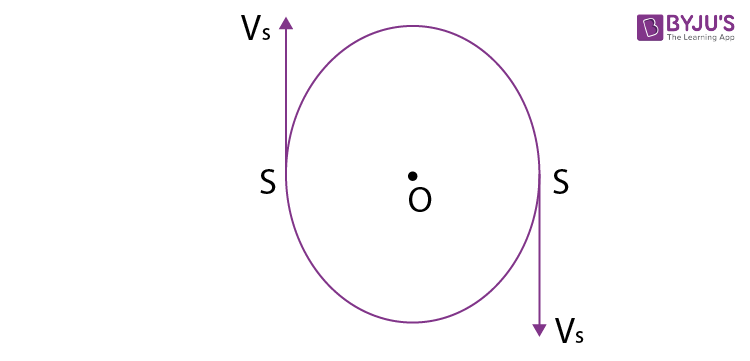2. When the observer is rotating:

When the observer moves towards the source then the frequency heard is maximum and is given by

$$\begin{array}{l}{{F}_{\max }}=\left( \frac{V+Vo}{V} \right)Fo\end{array}$$

When the observer moves away from the source, then the frequency heard is minimum and is given by

$$\begin{array}{l}{{F}_{\min }}=\left( \frac{V-Vo}{V} \right)Fo\end{array}$$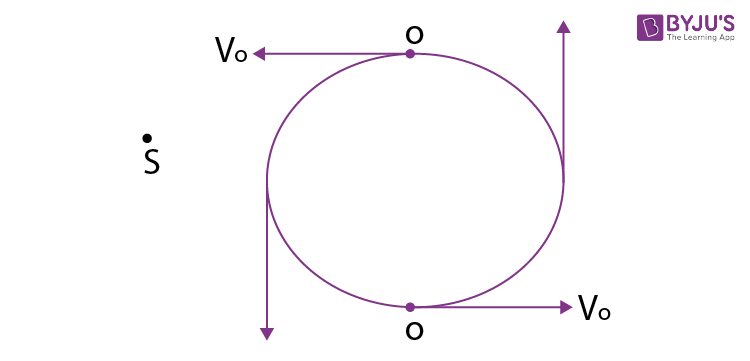When the source is at the centre of rotation then there will be no change in the frequency of the sound heard.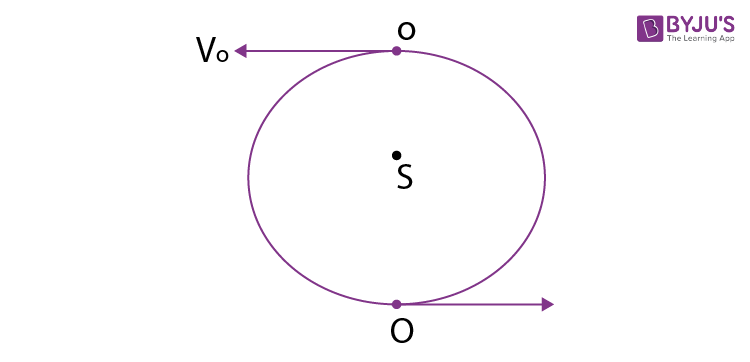### Limitations of Doppler Effect

• This effect can occur only when the velocities of the sound source and the observer are comparatively lower than the velocity of sound.
• Another set condition for this effect is that the motion of both source and the observer has to be along the same straight line.

## Inverse Doppler Effect

The possibility of an inverse Doppler effect has been speculated by many scientists such as Victor Veselago and others since 1968. Usually, the doppler shift size depends on the refractive index of the medium that the wave is moving or travelling through. However, it has been known that some materials have negative refraction. Now, with such property, there is a possibility of a Doppler shift that could work in a direction opposite to that of a conventional Doppler shift.

As such the first experiment in which the inverse Doppler effect was observed in 2003. It was conducted by Nigel Seddon and Trevor Bearpark in Bristol, United Kingdom. As more developments unfolded the inverse Doppler effect was also observed in some inhomogeneous materials.

## Application of Doppler Effect

Some of the common applications of the Doppler effect include;

• Astronomy: It is used to measure the speed at which stars and galaxies are approaching or receding from the earth.
• Radars: It is used to measure the velocity of detected objects as it approaches or recedes from the radar source.
• Sirens: Usually the siren slides as it approaches you so that it doesn’t hit you hard and loud.
• Satellites: It is used in exploited for satellite navigation such as in the case of Transit and DORIS. It is also used in satellite communication.
• Audio: Used in producing rapidly fluctuating frequencies of sound.
• Vibration measurement: Used mostly in a laser Doppler vibrometer (LDV).
• Medical imaging and blood flow management: This effect is widely used in Doppler ultrasonography in devices such as echocardiogram. It is basically used in velocity profile management wherein the phase shift is measured.
• Flow Measurement: Devices such as the Laser Doppler velocimeter (LDV) and acoustic Doppler velocimeter (ADV) which are based on the doppler effect are used to measure velocities in a fluid flow.

## Doppler Effect Problems Solved

#### Doppler Effect JEE Problems and Solutions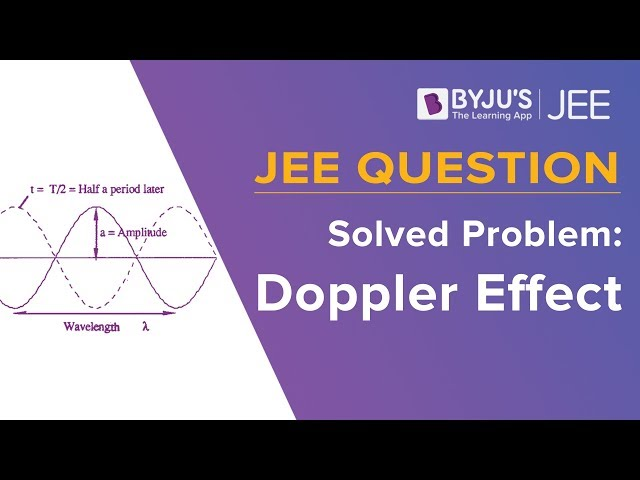## Frequently Asked Questions on Doppler Effect

### What is Doppler effect?

The change in the frequency of waves whenever there is a relative motion between the source of waves and the listener or observer is known as Doppler’s effect.

### Why does the whistle from the approaching engine appear to be shriller than that of the receding engine?

This is due to Doppler effect. Doppler effect states that if the source approaches the observer, the frequency of the sound increases and if the source of sound recedes from the observer, the frequency of sound decreases.

### What causes the Doppler effect when the listener is in motion?

When the listener is in motion, the velocity of the sound changes with respect to the observer, whereas the wavelength of the sound waves will remain the same.

### In which case is the Doppler effect not applicable?

When the source and the observer are at rest.

Test Your Knowledge on Doppler Effect